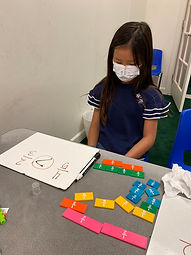## Ms. Kylie

### Target 1​

###### Lesson Type:

Continuation

Number Operation

:

Number Reltionships

Compare numbers, recording the results of the comparison with the symbol >, =, and <.

###### 1:

Compare fractions (proper and improper).

###### 2:

Compare decimals (up to the hundredths place).

###### 3:

When comparing decimals, understand to look at the greatest place-value first.

###### 4:

Explain how a number could have a lesser value, even if it has more digits than another (i.e., 4.33<5.2).

4th

###### Vocabulary:

Whole number, Tenths, Hundredths, Thousandths, Greater than, Less than, Equal to

Activities:

-Students practiced comparing decimal numbers using the greater than, less than and equal to symbol. They were given task cards with two numbers to compare or three decimal numbers to order from least to greatest or vice versa.

-Students played a game of comparing decimal numbers by each rolling three dice to create the greatest decimal number possible. The player who was able to make the greatest number won that round.

-Students were each given 10 decimal numbers to order from greatest to least.

-Students practiced comparing fractions using fraction bars to help them understand which fraction is greater or less.### Home Exploration

###### Guiding Questions:## Absent Students:

### Target 2

:

###### Vocabulary:

Activities:### Home Exploration

###### Guiding Questions:### Target 3

:

###### Vocabulary:

Activities:### Home Exploration